## Introduction

An algebraic equation is a mathematical statement with ‘=’ (equality) sign. It will have an algebraic statement with a variable on one side of the ‘=’ and a constant on the other side. Equations are applied in a variety of situations. Watch the video to learn more:

Did you know, you can use equations to make predictions about the future?For example, you are organising a bake sale in your school that is to last 5 days. You have already spent ₹7500 in procuring all the ingredients and tools required for the sale. Per day, you are generating revenues of ₹2500. To calculate the profit you make after 5 days, you can form a simple equation. Let the profit be x:

x = 2500 × 5 – 7500 = ₹5000

Using the equation, we can predict what the profit will be well ahead of the sales.

#### Concepts

The chapter ‘Simple Equations’ covers the following concepts:

#### Equations

Rashika is at a boutique. She is buying some dresses for herself.The cost of a pair of pants is ₹125 more than the cost of a t-shirt.So, which is more expensive? The pants or the t-shirt? The pants are more expensive. It is also given that:How can we write this using an algebraic statement? We can write it asIf the cost of a t-shirt is ‘₹x,’ then we can sayWhat do you notice in this statement?

• On each side of the ‘=’, we have a quantity and an expression.

Such statements, with an algebraic expression on one side and a constant or a variable on the other side of an ‘=’ sign, are called equations. An equation shows that two quantities are equal.

• The expression on the left of the ‘=’ is called the Left-Hand Side or LHS of the equation.
• The expression on the right of the ‘=’ is called the Right-Hand Side or RHS of the equation.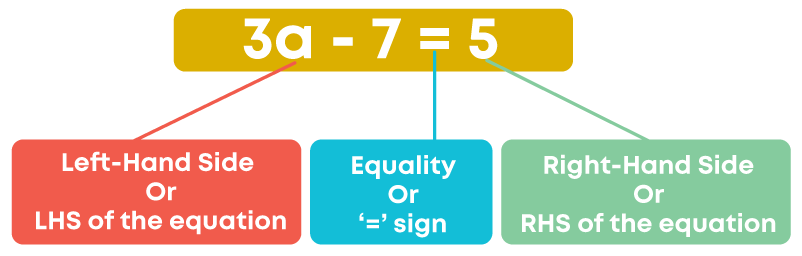Examples of equations are given below: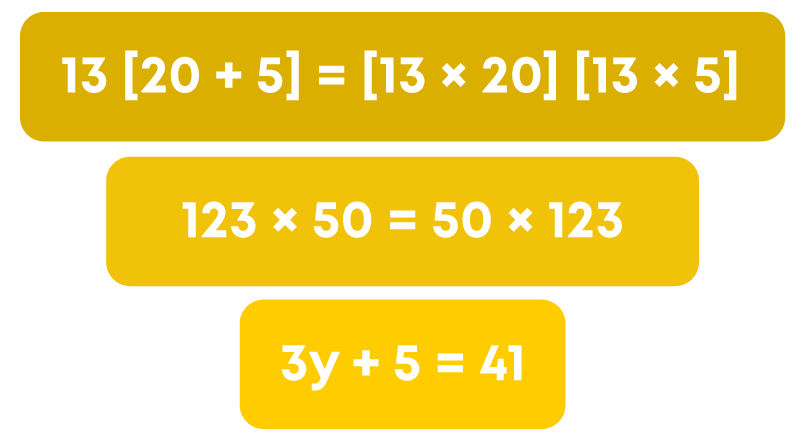How to write an equation from a given statement? When converting a statement into an equation

• Identify the unknown quantity.
• Identify the relation between the unknown quantity and known quantity.
• Identify the mathematical operation to be used.
• ‘added’ or ‘sum of’ or ‘increased by’ or ‘more than’ – All mean addition.
• ‘minus’ ‘difference’ ‘decreased by’ ‘less than’ – All mean subtraction.
• ‘multiplied by’ , ‘times’ , ‘of’ – All mean multiplication.
• ‘quotient ’ ‘divided by’ – All mean division.
• ‘is’ or ‘equals’ or ‘gives’ or ‘same as’ – All mean ‘equal to’ (‘=‘)

Beena tells Manish that she has a question to solve.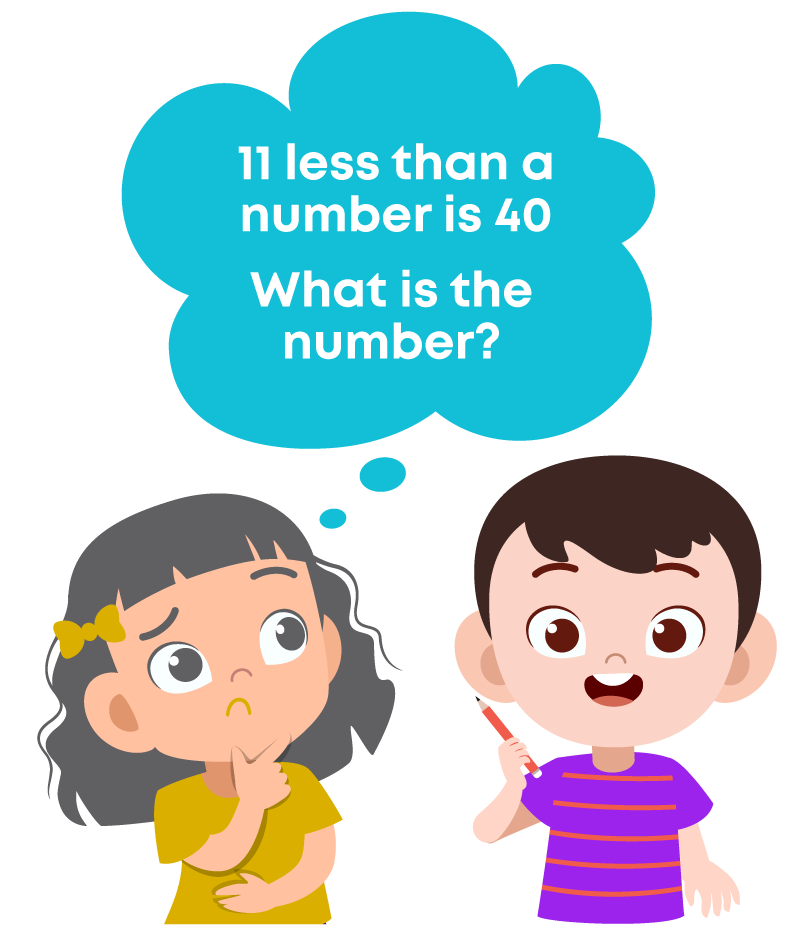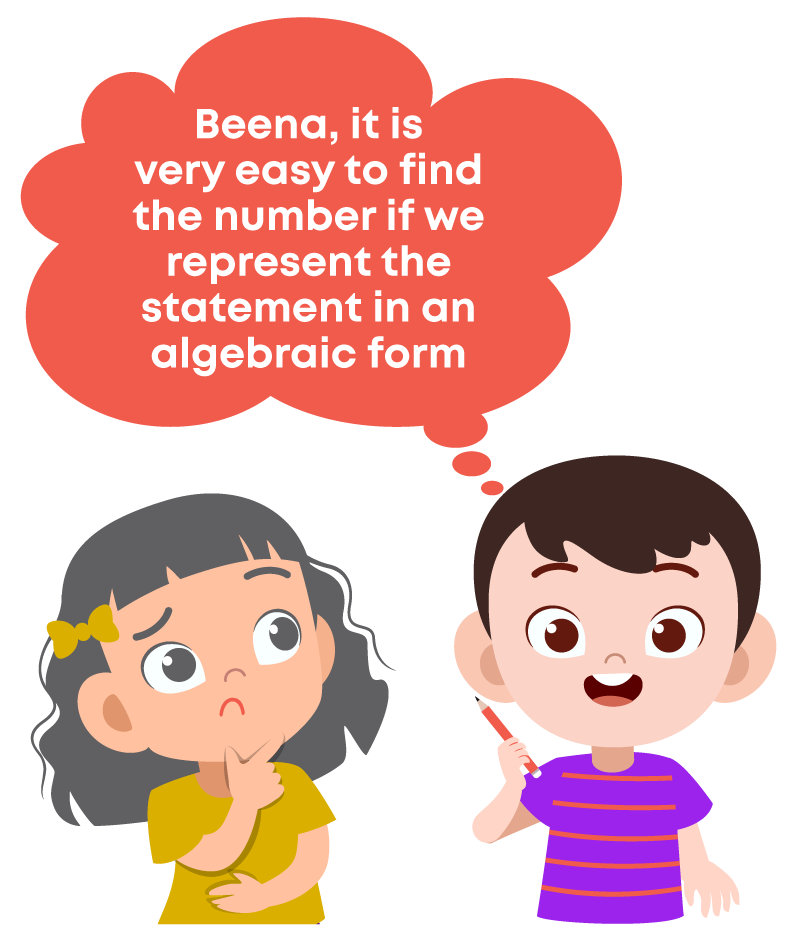Let us look at the statement again: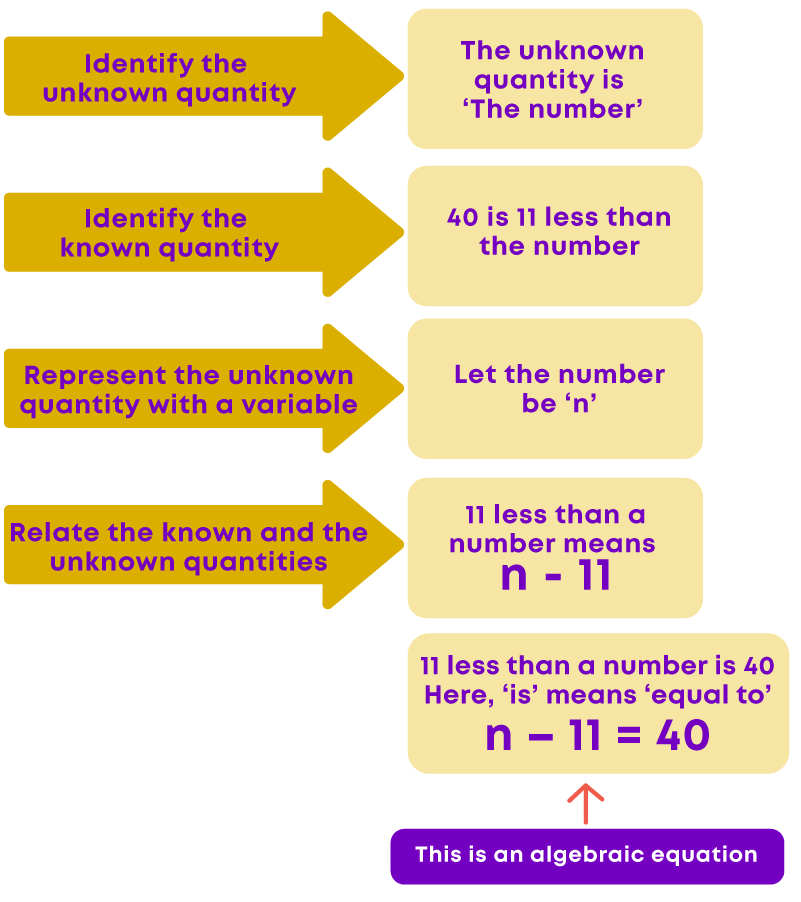Any expression can be represented in statement form. We can form different statements which mean the same for the same equation.

Consider the equation:Let us translate this equation to statement form.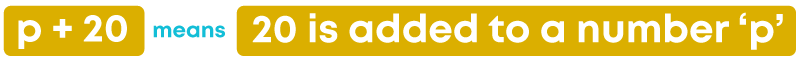So, the statement will be:What do you understand by the word ‘gives’? ‘Gives’ means ‘results in’ or ‘is equal to.’ ‘Gives’ can also be written as ‘equals’ or ‘results in’ or ‘is.’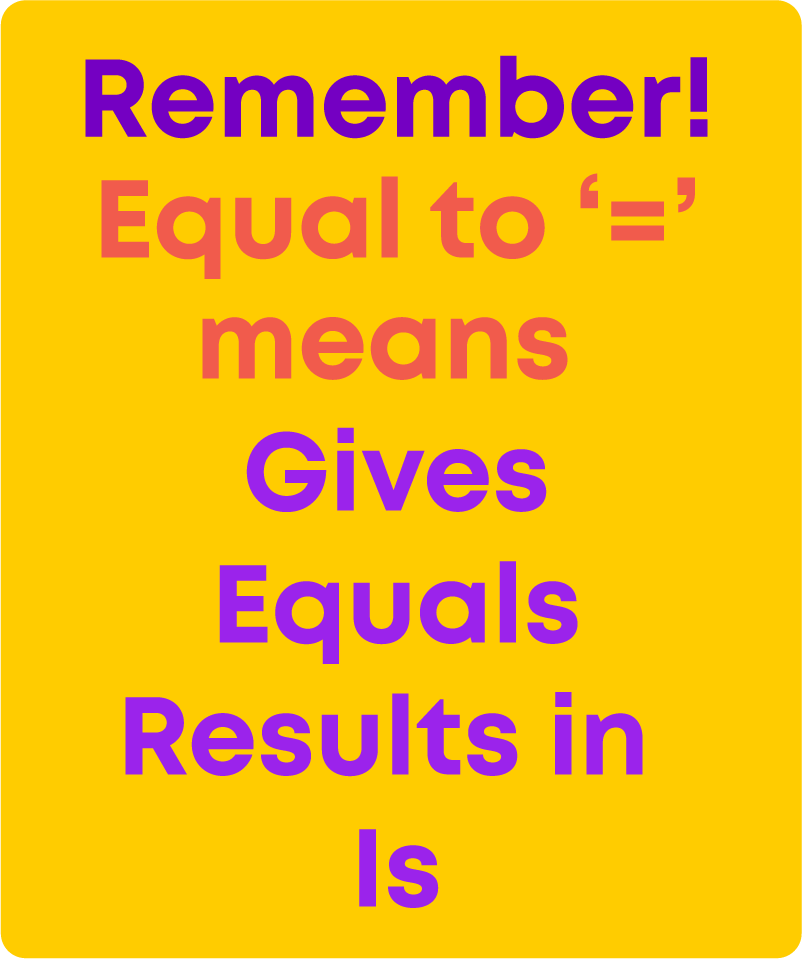You can use any of the words above if you have to represent ‘=‘ in word form.Consider the equation given here:Let us write a statement for this equation.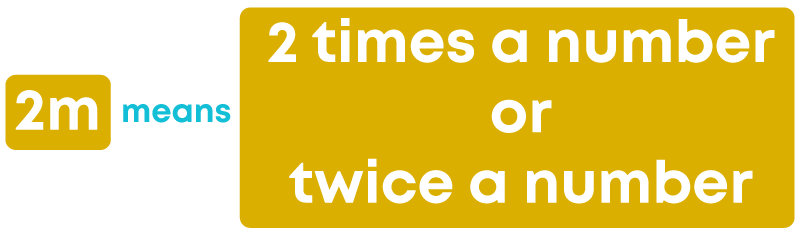Now, let us write a statement for this.What does ‘2n’ mean? ‘2n’ means ‘2 × n’

It also means ‘Two times a number’ or ‘a number multiplied by 2’.

Similarly,

‘5b’ means ‘5 times a number’ or ‘a number multiplied by 5’.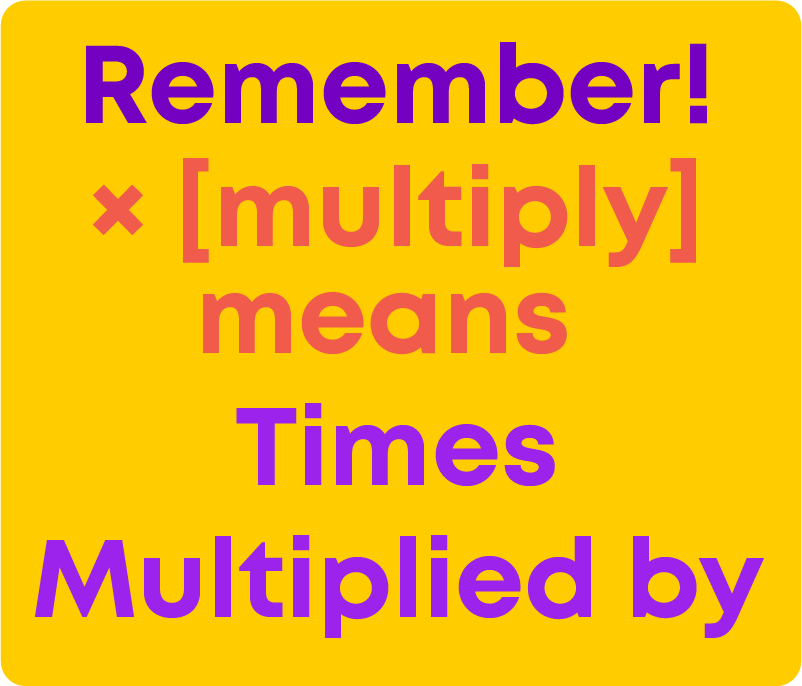Consider the following equation.Let us write this in statement form. What is on the left-hand side of the equation?

It is y – 11

This shows that 11 is subtracted from a number ‘y’. Now, the right-hand side has the number -3

y – 11 is equal to -3

So, the statement will beLet us see another kind of equation: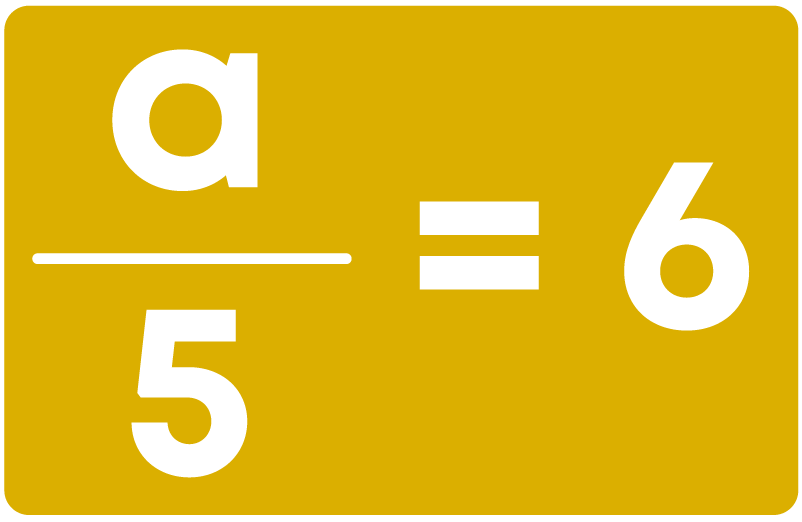Here,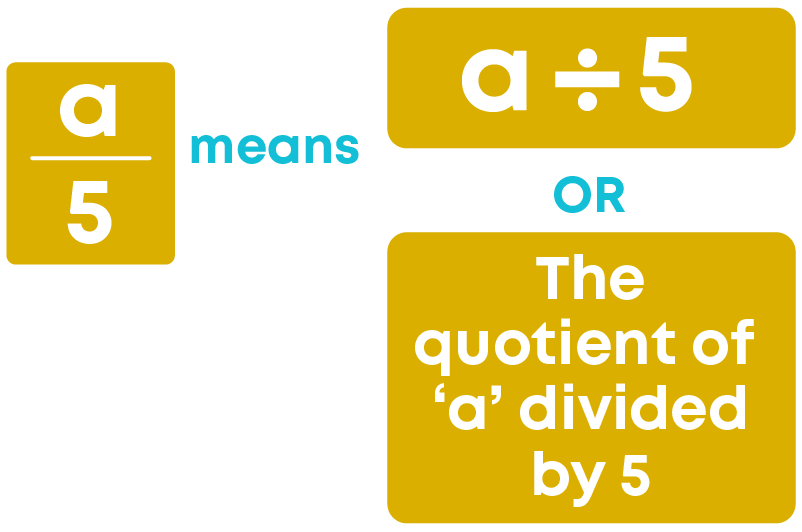So, the statement for the equation is: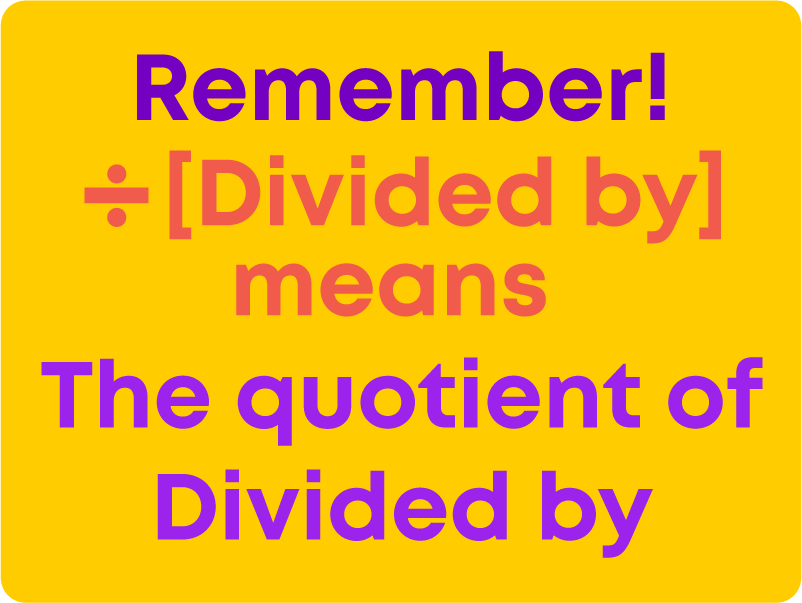#### Solving an Equation by Balancing Method

Balancing an equation is like weighing balance. On adding weight to only one side of a weighing balance, it gets disturbed.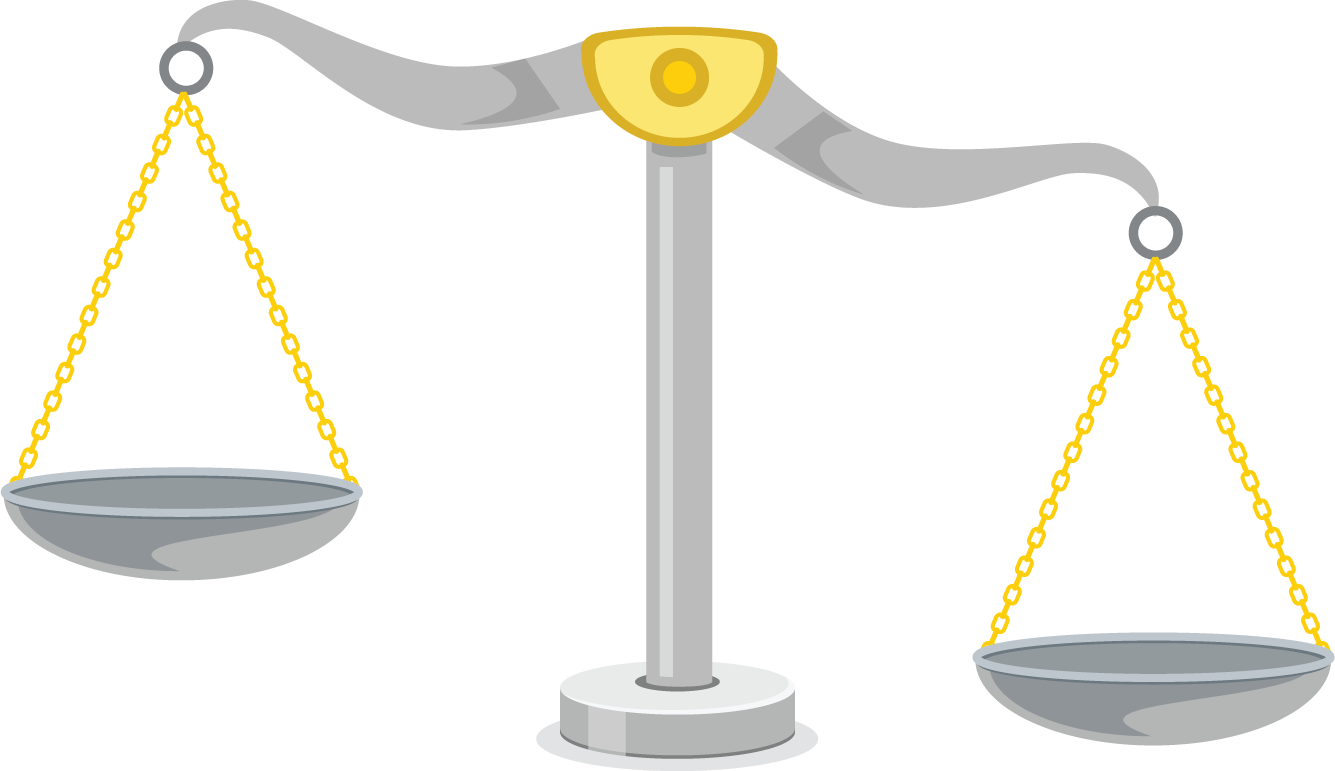By adding equal weight on the other side, balance is achieved.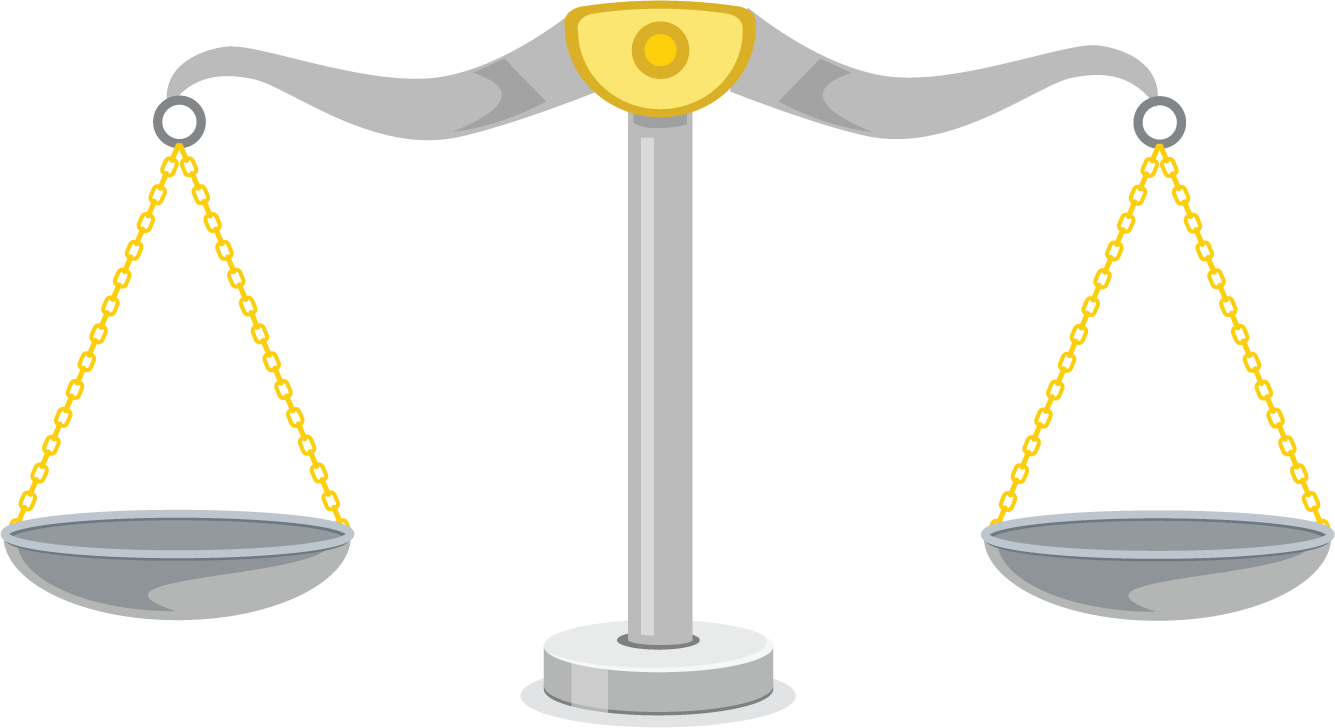If an operation is performed on only one side of an equation, then the balance of the equation is disturbed.Whatever operation is performed on one side of an equation, the same operation should be performed on the other side too.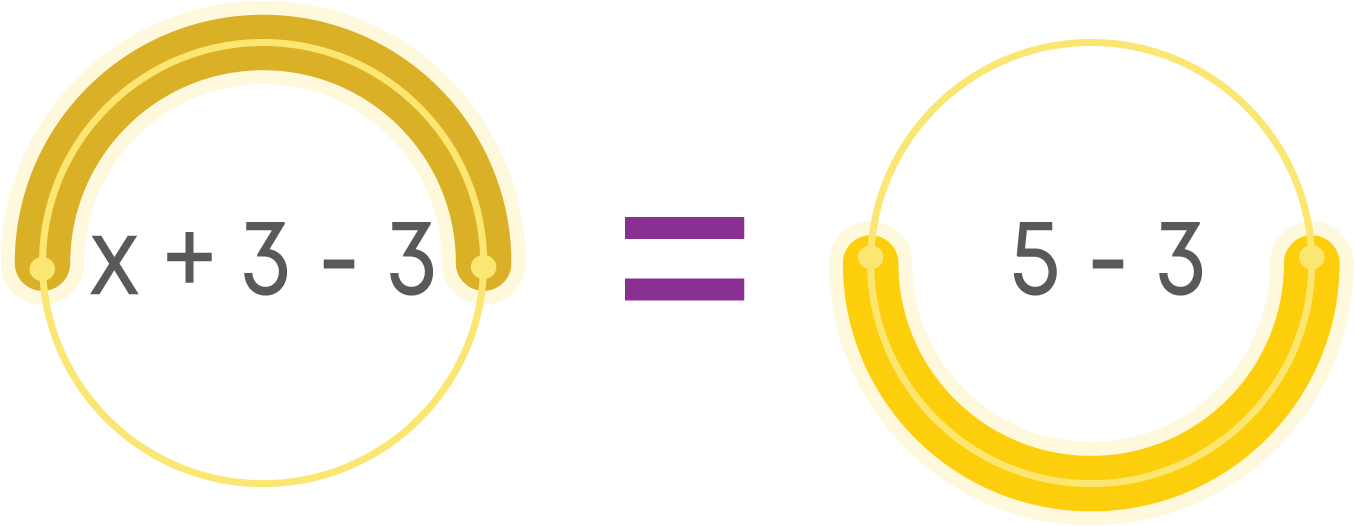Now, let us learn how an equation is balanced with addition and subtraction operations.

• To keep only the variable term on one side of an equation, eliminate constant terms.
• To eliminate constant, perform the opposite operation with the same constant.
• The equation is balanced only when the same quantity is added to/subtracted from both sides.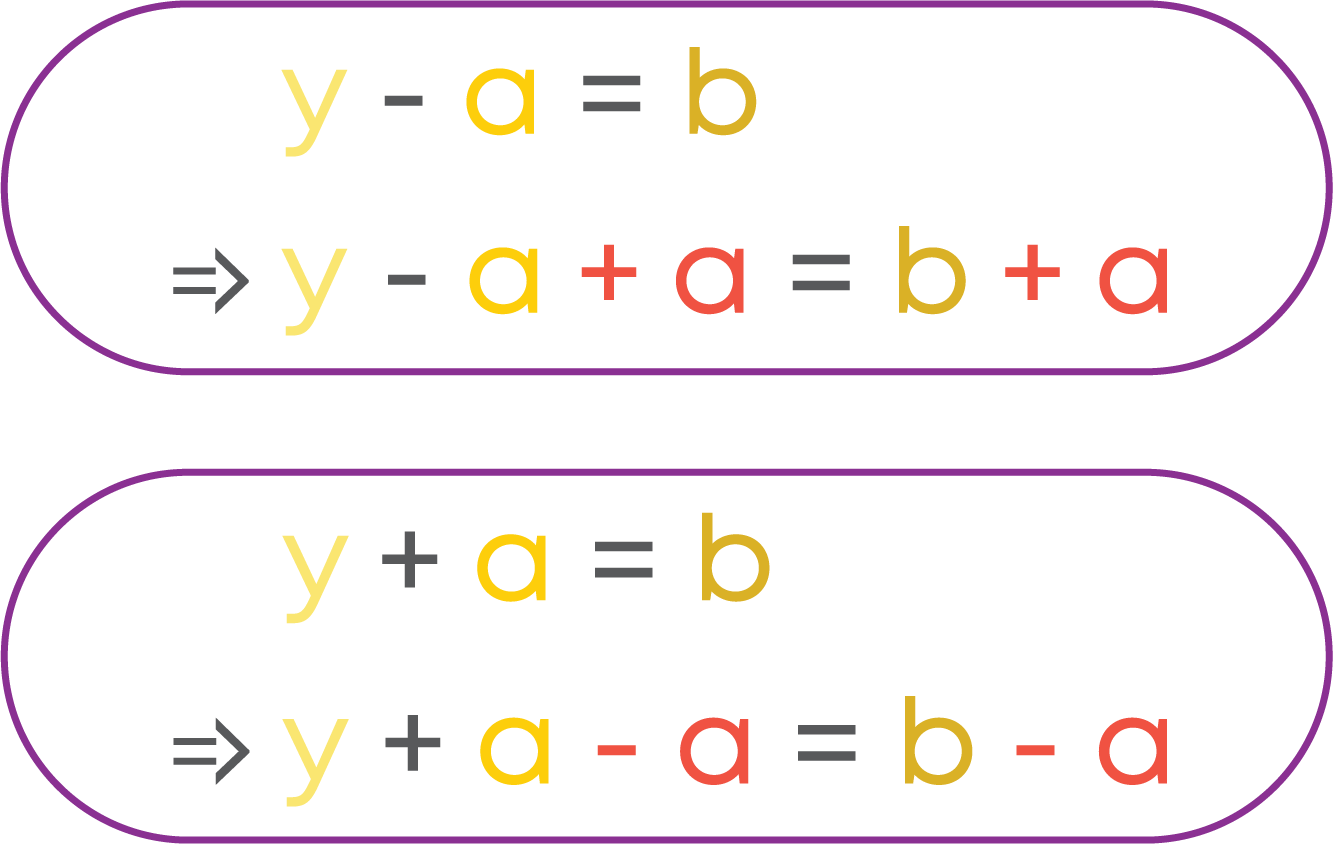To balance an equation with multiplication, we follow the following steps:

• To keep only variable term on one side of an equation, eliminate constant terms.
• To eliminate the constant, perform the opposite operation with the same constant.
• The equation is balanced only when both the sides are multiplied by the same quantity.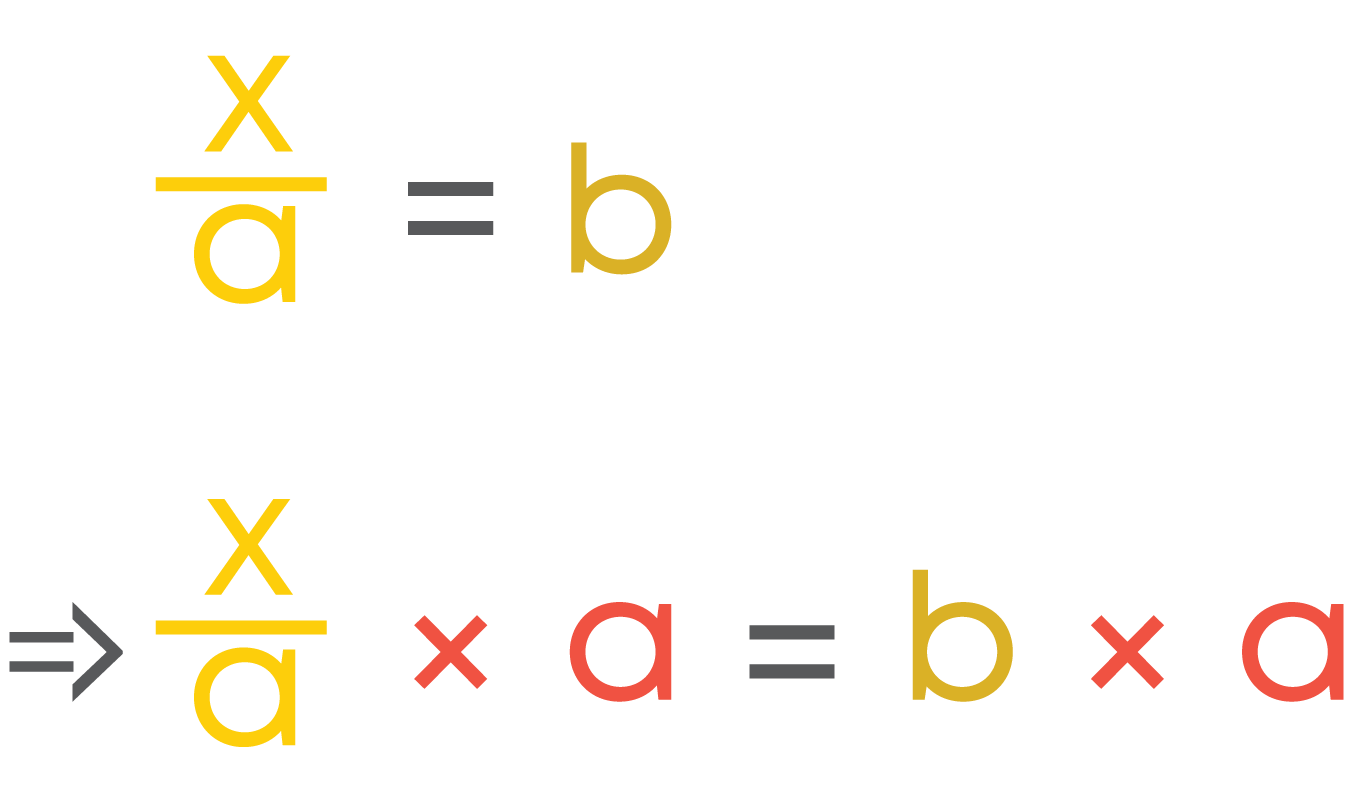To balance an equation with division, we follow the following steps:

• To keep only variable term on one side of an equation, eliminate constant terms.
• To eliminate the constant, perform the opposite operation with the same constant.
• The equation is balanced only when both the sides are divided by the same quantity.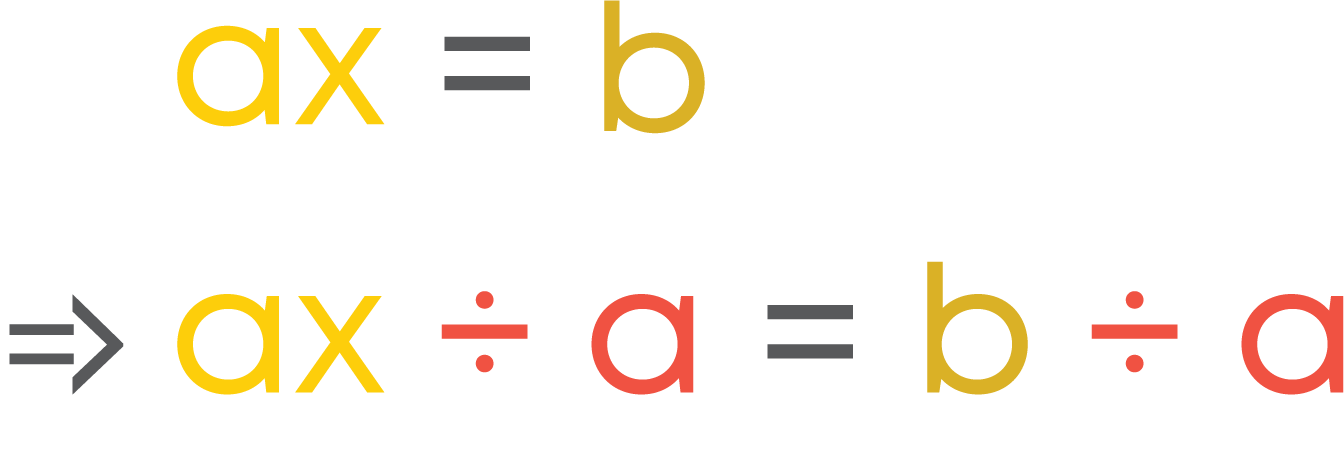We can solve equations by performing the same operation on both sides of the ‘=’ sign. This method is called the Balancing Method.

While solving an equation by Balance method:

• Our goal is to have only the variable of one side and the constants on the other side.
• This means we eliminate the constants on the side which has a variable.

To eliminate the constants / numbers from the side having the variable term, we should follow the steps given below:

• If there is a number added on the side having the variable term, we subtract the number from both sides.
• If there is a number subtracted on the side having the variable term, we add the number to both sides.
• If there is a number multiplied on the side having the variable term, we divide both the sides with the number.
• If there is a number that is divided, then we multiply both the sides with the number.

#### Solving an Equation by Transpose Method

We observed that while solving the equation y - 3 = 5 using the balancing method, we get y = 5 + 3.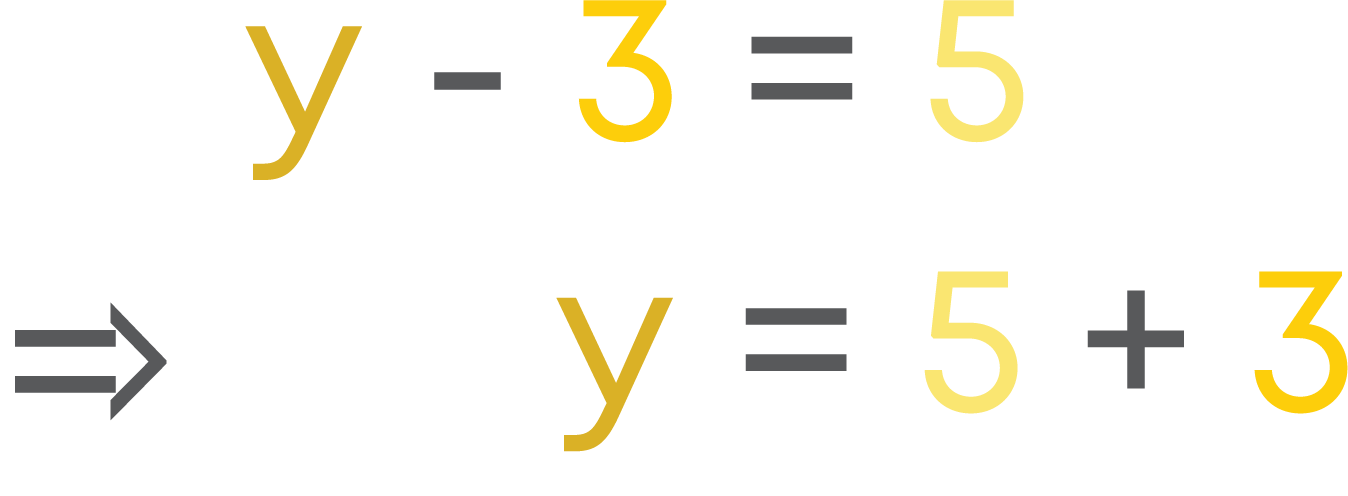That is, 3 is moved from the LHS to the RHS by changing the operation. This is called transposing.

It means that adding 3 to both sides is equivalent to transposing 3 from one side to the other side by changing the operation to subtraction.

So here, instead of adding 3 on both sides, we can directly transpose 3 to the other side with the opposite operation.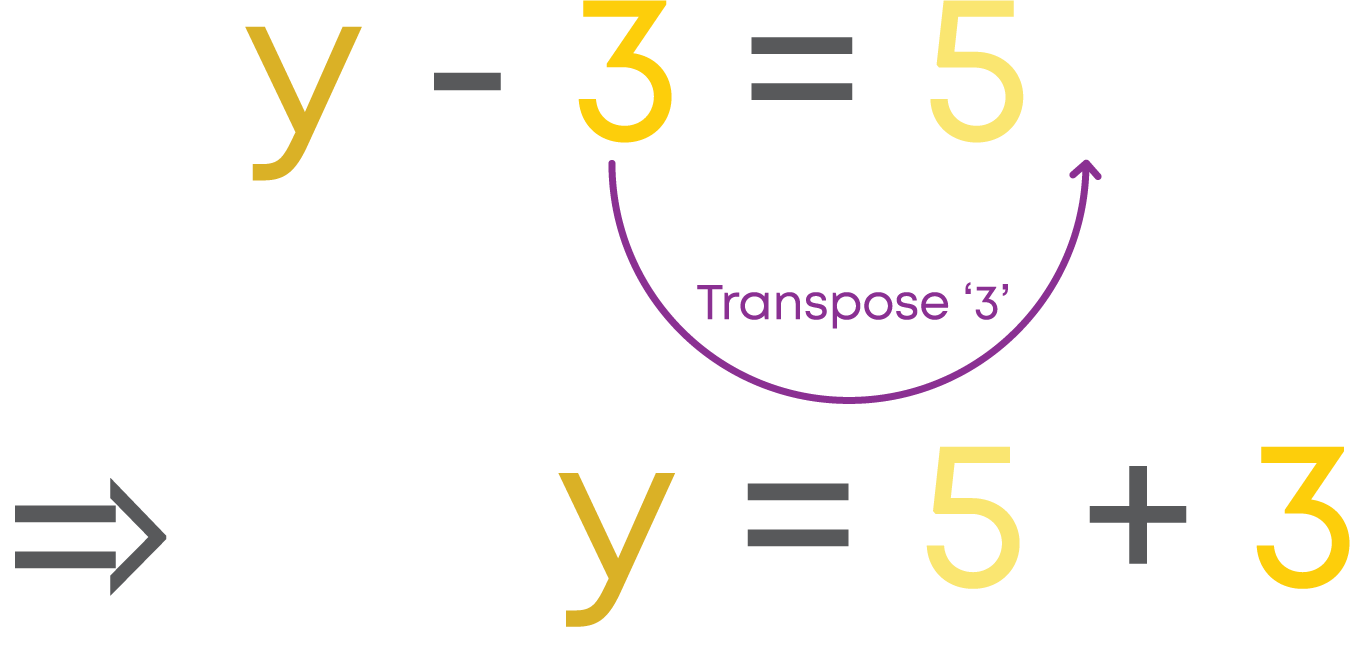Transposing is a shortcut of balancing.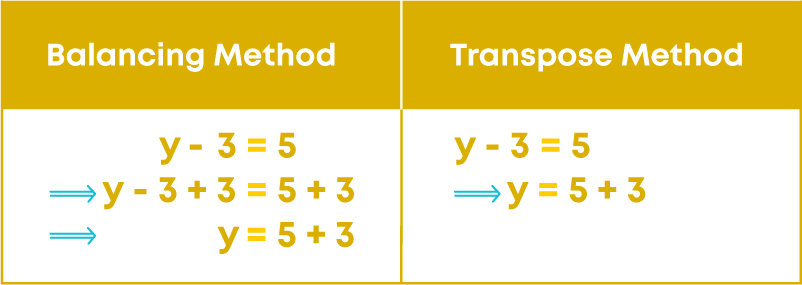Transposing is equivalent to adding/subtracting/multiplying/dividing on both sides.

Adding/ subtracting a term on both sides of an equation is equivalent to transposing the term from one side to the other side of the equation by interchanging the operations subtraction and addition.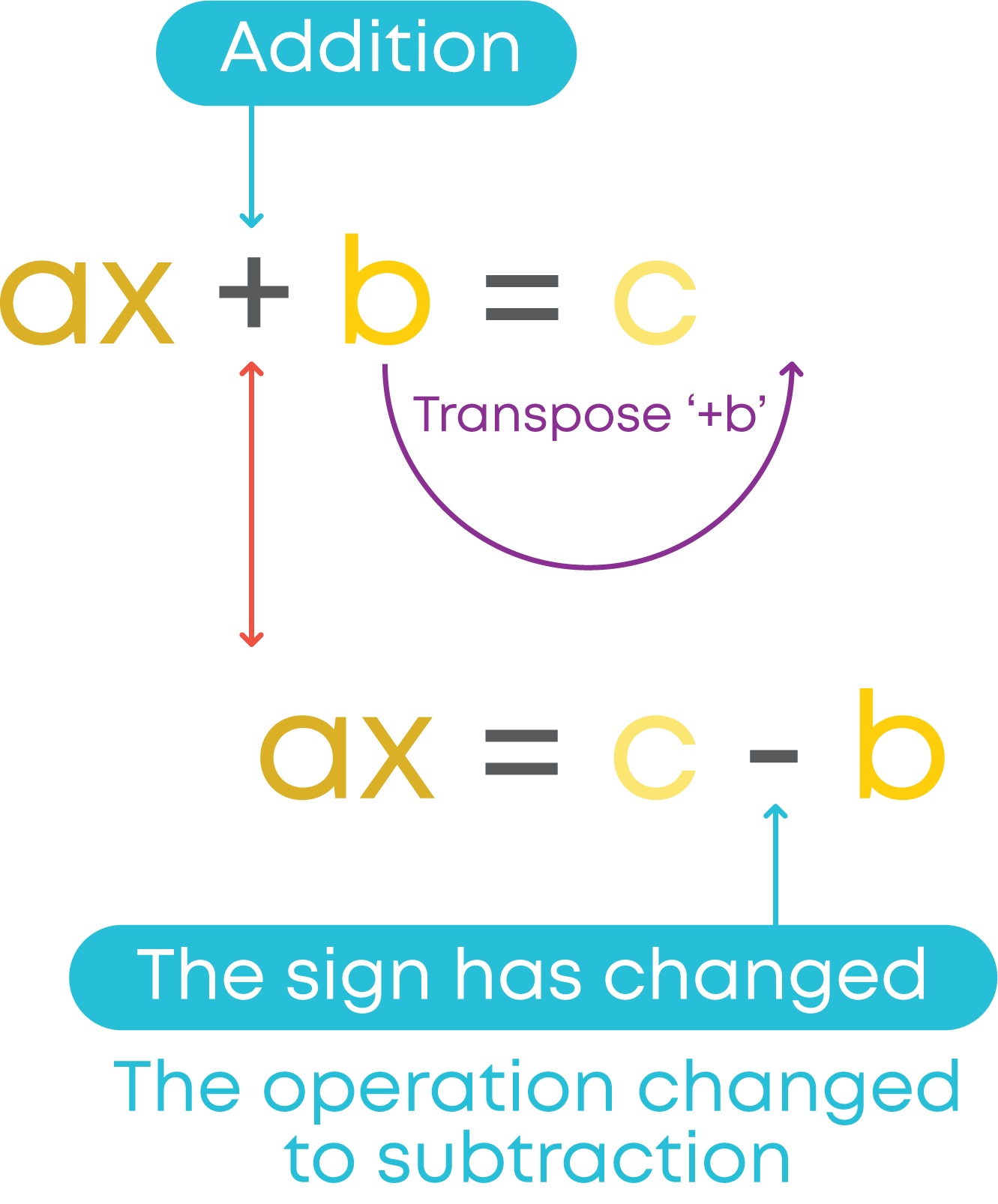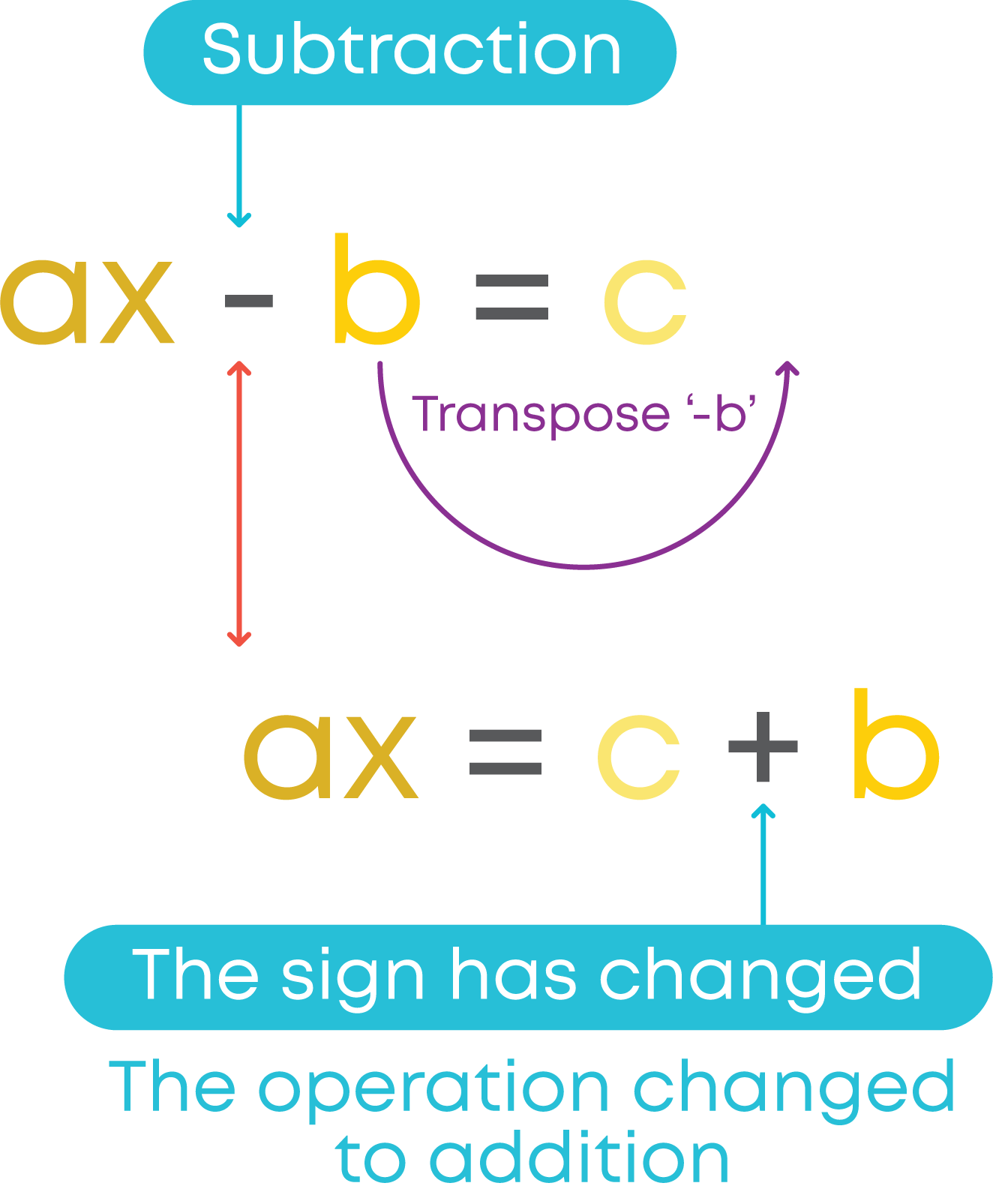Multiplying/ dividing a term on both sides of an equation is equivalent to transposing the term from one side to the other side of the equation by interchanging the operations multiplication and division.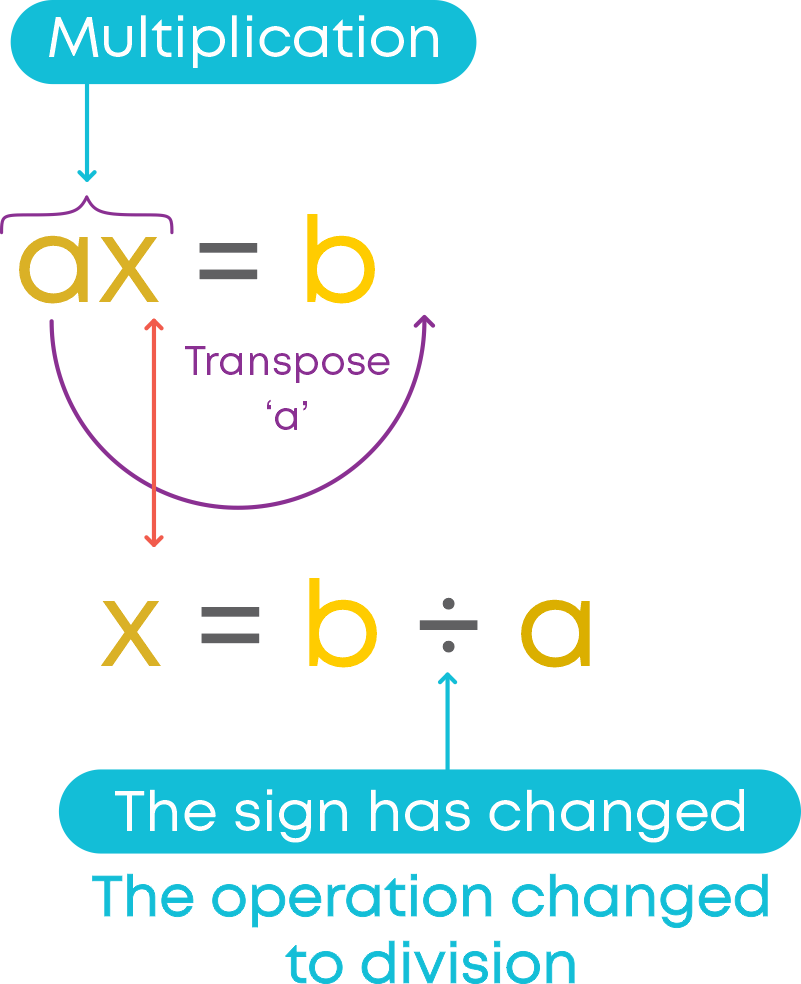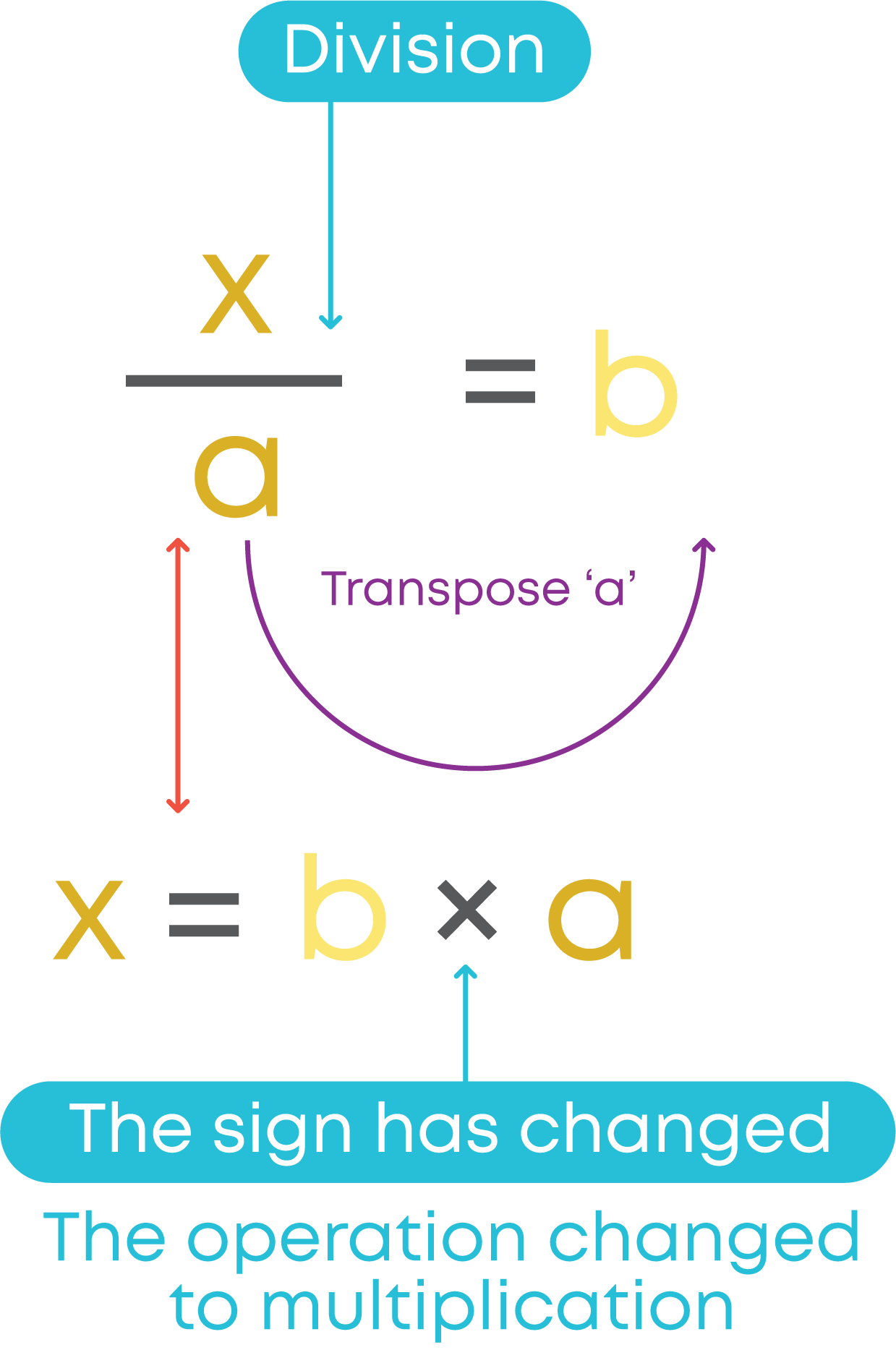Consider the equation is x - 8 = 2. Let us solve the given equation using the transpose method.

To get only the variable on the LHS of the equation, we transpose 8. On transposing, we perform the opposite operation. On the LHS, 8 is subtracted from ‘x’.

So, on the RHS it will be added to 2. Hence, we get,

x = 2 + 8

⇒ x = 10

Hence, x = 10

Now, consider the equation -4y - 7 = 0

Solution of this equation is y = -7 4

Can it be the solution of any other equation?

To understand this, we go in the reverse way.

Consider y = -7 4

• Multiply both sides by 4 to get 4y = -7.
• Add 1 to both sides to get 4y + 1 = -6.

Thus, 4y + 1 = -6 is an equation with the solution y = -7 4

Similarly, we can find many equations for which y = -7 4 can be the solution.

Those equations are constructed starting with y = -7 4

From a given solution, equations can be constructed by performing the same operations on both sides using the same number.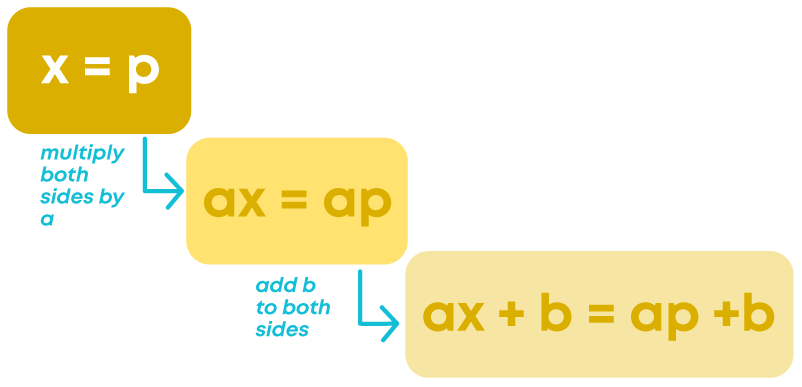Operations can be chosen in any order/combination. Any number can be chosen to perform operations.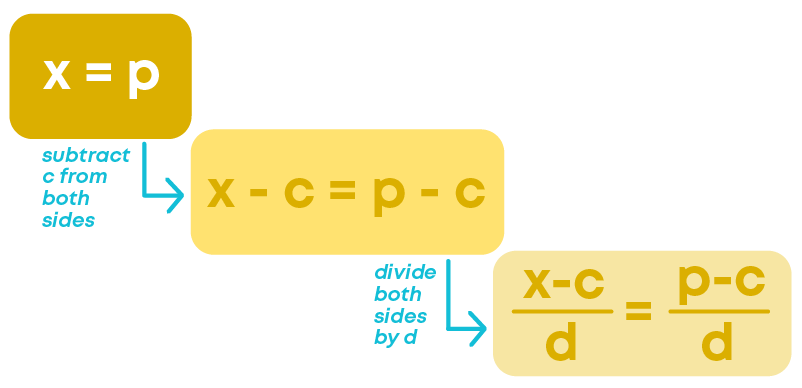#### Common Errors

The following are topics in which students make common mistakes when dealing with simple equations:

• 1. Solving an equation by Balance method
• 2. Solving an equation by Transpose method

#### Solving An Equation By Balance Method

While solving a linear equation by balance method, we have to add/subtract/multiply /divide by the same number on both sides to maintain the balance of the equation.

Example:

Consider the equation:To solve this equation, we need to subtract 2 from the LHS and RHS.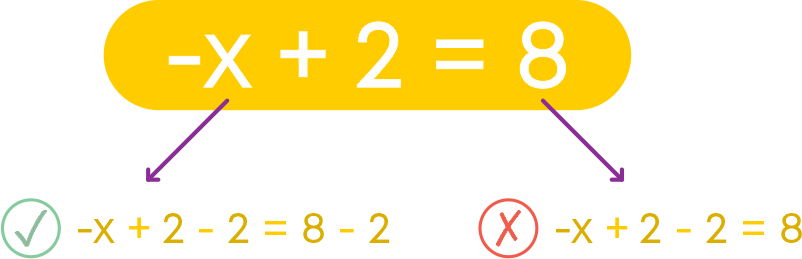#### Solving An Equation By Transpose Method

While solving a linear equation by transpose method, we have to transpose the constant terms from one side to another side until we have only the variable on one side and the constant on the other side.

When we transpose a constant to the opposite side, its operation changes to the opposite.

Example:

• +3 when transposed to the opposite side becomes -3
• -5 when transposed to the opposite side becomes +5
• A number multiplied with the variable on one side, becomes division when transposed to the other side.
• A number which divides the variable on one side becomes multiplication when transposed to the other side.

Example:

(1)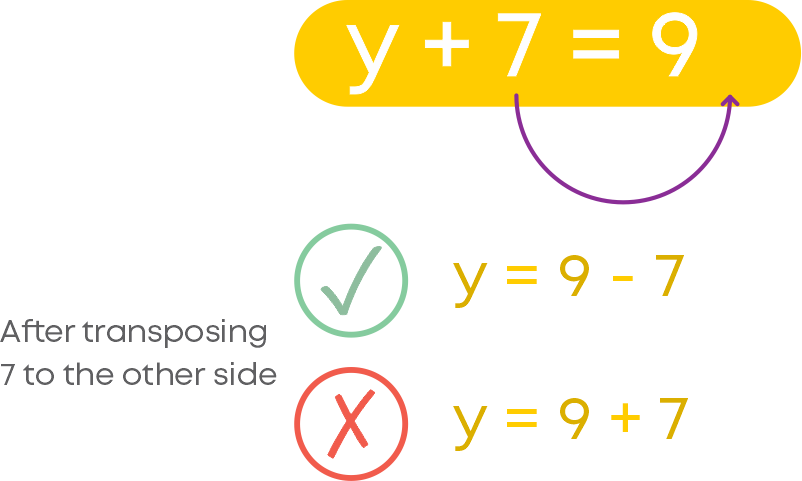(2)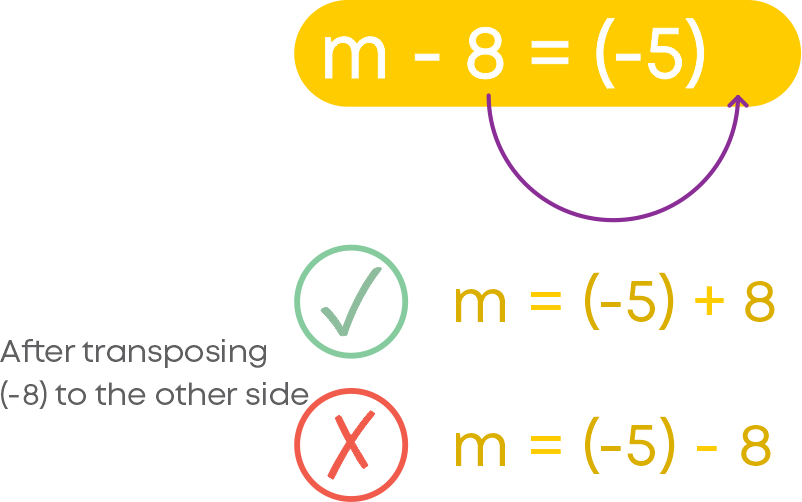(3)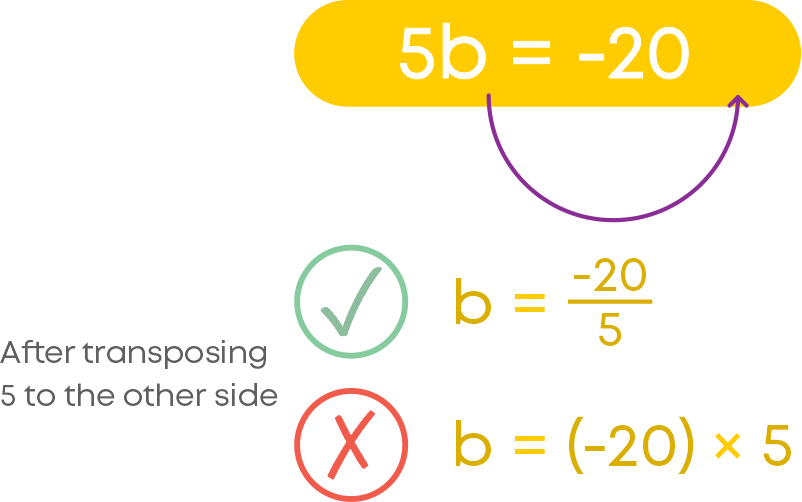(4)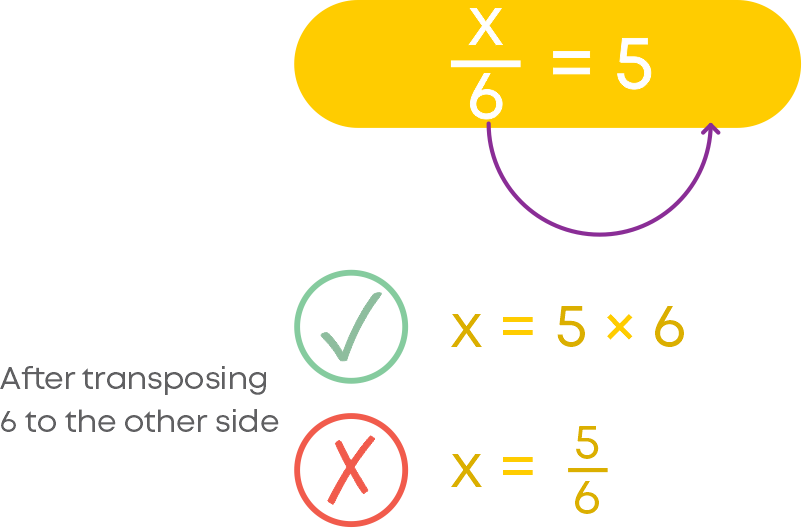#### Conclusion

You have learnt about equations and how to solve them with multiple operations involved. Can you solve the riddle below?

I am a three-digit number.

My second digit is 4 times bigger than the third digit.

My first digit is 3 less than my second digit.

Who am I?#### Arpana

Author

Arpana is an education specialist with years of teaching Math and developing content. She previously worked as a freelance content developer, developing lesson plans for a reputed publisher of text books and content for various educational companies. She is an enthusiastic teacher who has taught students from India, the UK, and New Zealand.